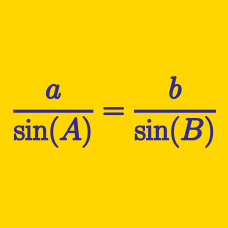Geometry

# Sine Rule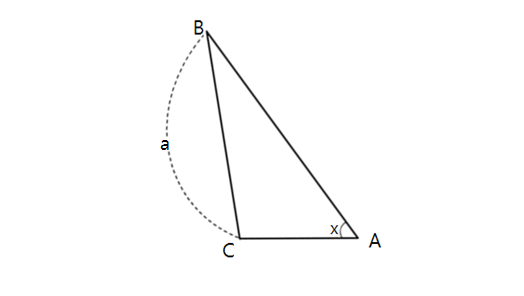In triangle $$ABC$$ above, $\angle BAC =x= 30^{\circ}, \sin \angle ABC = \frac{1}{4}, \lvert \overline{BC} \rvert =a= 9,$ where $$\lvert \overline{BC} \rvert$$ is the length of $$\overline{BC}.$$ What is the value of $$\lvert \overline{AC} \rvert^2?$$

Triangle $$ABC$$ has angles $$\angle A = 60^{\circ}$$ and $$\angle B = 45^{\circ}$$, and a side length $$a = 21 \sqrt{6}$$. What is the side length $$b$$?

Details and assumptions

$$a$$ and $$b$$ are the lengths of the sides opposite to the vertices $$A$$ and $$B$$, respectively.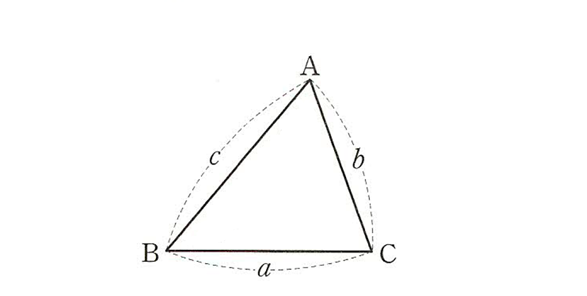In triangle $$ABC$$ above, we are given the side lengths as follows:$\lvert\overline{BC}\rvert=a, \lvert\overline{CA}\rvert=b, \lvert\overline{AB}\rvert=c.$ If $$ab : bc : ca = 2 : 11 : 5,$$ what is the value of $\frac{\sin A + \sin B}{\sin C}?$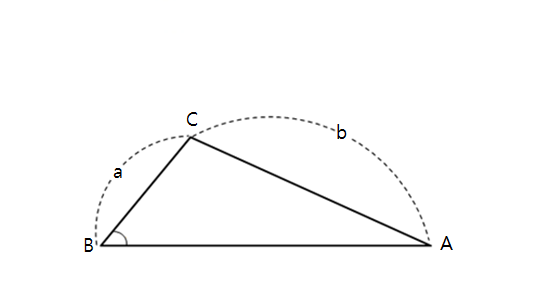In triangle $$ABC$$ above, $$\angle ABC =x= 30^{\circ}$$ and $\lvert \overline{BC} \rvert =a= 6, \lvert \overline{CA} \rvert =b= 10.$ What is the value of $$\left(\sin \angle BAC\right)^2?$$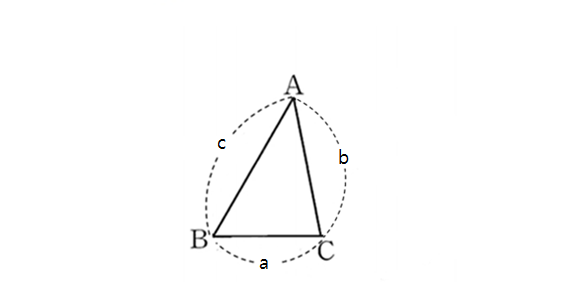In triangle $$ABC$$ above, we are given the side lengths as follows:$\lvert\overline{BC}\rvert=a, \lvert\overline{CA}\rvert=b, \lvert\overline{AB}\rvert=c.$ If $$\sin A : \sin B = 5 : 4$$ and $$\ a : c = 2 : 5,$$ what is $$a : b : c?$$

Note: The above diagram is not drawn to scale.

×

Problem Loading...

Note Loading...

Set Loading...